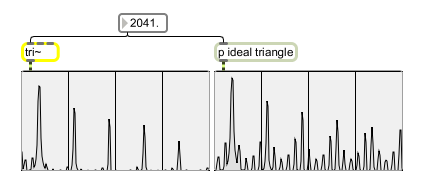# tri~

Antialiased triangular oscillator

## Description

tri~ generates a triangle wave whose component frequencies are resistant to aliasing.

## Arguments

Name Type Opt Description
initial-frequency (Hz) int or float First argument sets the initial frequency of the oscillator. The default is 0. Second argument sets the duty cycle. The default is 0.5.

## Messages

 int frequency (Hz) [int] In left inlet: Sets the frequency of the oscillator. In middle inlet: Sets the duty cycle of the oscillator. Signal is wrapped into the range 0-1. A value of 0.5 will produce a triangular wave that spends equal amounts of time sloping positively and negatively. float frequency (Hz) [float] In left inlet: Sets the frequency of the oscillator. In middle inlet: Sets the duty cycle of the oscillator. Signal is wrapped into the range 0-1. A value of 0.5 will produce a triangular wave that spends equal amounts of time sloping positively and negatively. signal In left inlet: Sets the frequency of the oscillator. In middle inlet: Sets the duty cycle of the oscillator. Signal is wrapped into the range 0-1. A value of 0.5 will produce a triangular wave that spends equal amounts of time sloping positively and negatively. In right inlet: (optional) A sync signal. When the control signal crosses from below 0.5 to above 0.5, the oscillator resets itself. A phasor~ object works well for this purpose. The classic use is to "sweep" this control signal in a frequency range somewhere at least three or four octaves higher than the fundamental frequency.

## Output

signal: An antialiased triangular waveform. An ideal, straight-line triangular wave generated in a computer contains aliased frequencies that can sound irritating. tri~ produces a nice, analog-esque output waveform.

## ExamplesSpectral comparison of tri~ and an ideal triangular wave